# Combinatorics

Combinatorics

Combinatorics is a branch of mathematics concerning the study of finite or countable discrete structures. Aspects of combinatorics include counting the structures of a given kind and size (enumerative combinatorics), deciding when certain criteria can be met, and constructing and analyzing objects meeting the criteria (as in combinatorial designs and matroid theory), finding "largest", "smallest", or "optimal" objects (extremal combinatorics and combinatorial optimization), and studying combinatorial structures arising in an algebraic context, or applying algebraic techniques to combinatorial problems (algebraic combinatorics).

Combinatorial problems arise in many areas of pure mathematics, notably in algebra, probability theory, topology, and geometry, and combinatorics also has many applications in optimization, computer science, ergodic theory and statistical physics. Many combinatorial questions have historically been considered in isolation, giving an ad hoc solution to a problem arising in some mathematical context. In the later twentieth century, however, powerful and general theoretical methods were developed, making combinatorics into an independent branch of mathematics in its own right. One of the oldest and most accessible parts of combinatorics is graph theory, which also has numerous natural connections to other areas. Combinatorics is used frequently in computer science to obtain formulas and estimates in the analysis of algorithms.

A mathematician who studies combinatorics is called a combinatorialist.

## History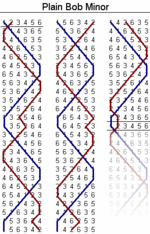An example of change ringing (with six bells), one of the earliest nontrivial results in Graph Theory.

Basic combinatorial concepts and enumerative results appeared throughout the ancient world. In 6th century BCE, physician Sushruta asserts in Sushruta Samhita that 63 combinations can be made out of 6 different tastes, taken one at a time, two at a time, etc., thus computing all 26-1 possibilities. Roman historian Plutarch discusses an argument between Chrysippus (3rd century BCE) and Hipparchus (2nd century BCE) of a rather delicate enumerative problem, which was later shown to be related to Schröder numbers. In the Ostomachion, Archimedes (3rd century BCE) considers a tiling puzzle.

In the Middle Ages, combinatorics continued to be studied, largely outside of the European civilization. Notably, an Indian mathematician Mahavira (c. 850) provided the general formulae for the number of permutations and combinations. The philosopher and astronomer Rabbi Abraham ibn Ezra (c. 1140) established the symmetry of binomial coefficients, while a closed formula was obtained later by the talmudist and mathematician Levi ben Gerson (better known as Gersonides), in 1321. The arithmetical triangle— a graphical diagram showing relationships among the binomial coefficients— was presented by mathematicians in treatises dating as far back as the 10th century, and would eventually become known as Pascal's triangle. Later, in Medieval England, campanology provided examples of what is now known as Hamiltonian cycles in certain Cayley graphs on permutations.

During the Renaissance, together with the rest of mathematics and the sciences, combinatorics enjoyed a rebirth. Works of Pascal, Newton, Jacob Bernoulli and Euler became foundational in the emerging field. In the modern times, the works by J. J. Sylvester (late 19th century) and Percy MacMahon (early 20th century) laid the foundation for enumerative and algebraic combinatorics. Graph theory also enjoyed an explosion of interest at the same time, especially in connection with the four color problem.

In the second half of 20th century, combinatorics enjoyed a rapid growth, which led to establishment of dozens of new journals and conferences in the subject. In part, the growth was spurred by new connections and applications to other fields, ranging from algebra to probability, from functional analysis to number theory, etc. These connections shed the boundaries between combinatorics and parts of mathematics and theoretical computer science, but at the same time led to a partial fragmentation of the field.

## Approaches and subfields of combinatorics

### Enumerative combinatorics

Enumerative combinatorics is the most classical area of combinatorics, and concentrates on counting the number of certain combinatorial objects. Although counting the number of elements in a set is a rather broad mathematical problem, many of the problems that arise in applications have a relatively simple combinatorial description. Fibonacci numbers is the basic example of a problem in enumerative combinatorics. The twelvefold way provides a unified framework for counting permutations, combinations and partitions.

### Analytic combinatorics

Analytic combinatorics concerns the enumeration of combinatorial structures using tools from complex analysis and probability theory. In contrast with enumerative combinatorics which uses explicit combinatorial formulae and generating functions to describe the results, analytic combinatorics aims at obtaining asymptotic formulae.

### Partition theory

Partition theory studies various enumeration and asymptotic problems related to integer partitions, and is closely related to q-series, special functions and orthogonal polynomials. Originally a part of number theory and analysis, it is now considered a part of combinatorics or an independent field. It incorporates the bijective approach and various tools in analysis, analytic number theory, and has connections with statistical mechanics.

### Graph theory

Graphs are basic objects in combinatorics. The questions range from counting (e.g., the number of graphs on n vertices with k edges) to structural (e.g., which graphs contain Hamiltonian cycles) to algebraic questions (e.g., given a graph G and two numbers x and y, does the Tutte polynomial TG(x,y) have a combinatorial interpretation?). It should be noted that while there are very strong connections between graph theory and combinatorics, these two are sometimes thought of as separate subjects.

### Design theory

Design theory is a study of combinatorial designs, which are collections of subsets with certain intersection properties. Block designs are combinatorial designs of a special type. This area is one of the oldest parts of combinatorics, such as in Kirkman's schoolgirl problem proposed in 1850. The solution of the problem is a special case of a Steiner system, which systems play an important role in the classification of finite simple groups. The area has further connections to coding theory and geometric combinatorics.

### Finite geometry

Finite geometry is the study of geometric systems having only a finite number of points. Structures analogous to those found in continuous geometries (Euclidean plane, real projective space, etc.) but defined combinatorially are the main items studied. This area provides a rich source of examples for Design theory. It should not be confused with Discrete geometry (Combinatorial geometry).

### Order theory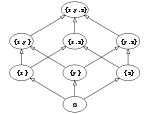Hasse diagram of the powerset of {x,y,z} ordered by inclusion.

Order theory is the study of partially ordered sets, both finite and infinite. Various examples of partial orders appear in algebra, geometry, number theory and throughout combinatorics and graph theory. Notable classes and examples of partial orders include lattices and Boolean algebras.

### Matroid theory

Matroid theory abstracts part of geometry. It studies the properties of sets (usually, finite sets) of vectors in a vector space that do not depend on the particular coefficients in a linear dependence relation. Not only the structure but also enumerative properties belong to matroid theory. Matroid theory was introduced by Hassler Whitney and studied as a part of the order theory. It is now an independent field of study with a number of connections with other parts of combinatorics.

### Extremal combinatorics

Extremal combinatorics studies extremal questions on set systems. The types of questions addressed in this case are about the largest possible graph which satisfies certain properties. For example, the largest triangle-free graph on 2n vertices is a complete bipartite graph Kn,n. Often it is too hard even to find the extremal answer f(n) exactly and one can only give an asymptotic estimate.

Ramsey theory is another part of extremal combinatorics. It states that any sufficiently large configuration will contain some sort of order. It is an advanced generalization of the pigeonhole principle.

### Probabilistic combinatorics

In probabilistic combinatorics, the questions are of the following type: what is the probability of a certain property for a random discrete object, such as a random graph? For instance, what is the average number of triangles in a random graph? Probabilistic methods are also used to determine the existence of combinatorial objects with certain prescribed properties (for which explicit examples might be difficult to find), simply by observing that the probability of randomly selecting an object with those properties is greater than 0. This approach (often referred to as the probabilistic method) proved highly effective in applications to extremal combinatorics and graph theory. A closely related area is the study of finite Markov chains, especially on combinatorial objects. Here again probabilistic tools are used to estimate the mixing time.

Often associated with Paul Erdős, who did the pioneer work on the subject, probabilistic combinatorics was traditionally viewed as a set of tools to study problems in other parts of combinatorics. However, with the growth of applications to analysis of algorithms in computer science, as well as classical probability, additive and probabilistic number theory, the area recently grew to become an independent field of combinatorics.

### Algebraic combinatorics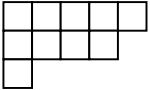Young diagram of a partition (5,4,1).

Algebraic combinatorics is an area of mathematics that employs methods of abstract algebra, notably group theory and representation theory, in various combinatorial contexts and, conversely, applies combinatorial techniques to problems in algebra. Within the last decade or so, algebraic combinatorics came to be seen more expansively as the area of mathematics where the interaction of combinatorial and algebraic methods is particularly strong and significant. One of the fastest developing subfields within algebraic combinatorics is combinatorial commutative algebra.

### Combinatorics on words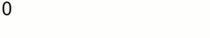Construction of a Thue-Morse infinite word.

Combinatorics on words deals with formal languages. It arose independently within several branches of mathematics, including number theory, group theory and probability. It has applications to enumerative combinatorics, fractal analysis, theoretical computer science, automata theory and linguistics. While many applications are new, the classical Chomsky–Schützenberger hierarchy of classes of formal grammars is perhaps the best known result in the field.

### Geometric combinatorics

Geometric combinatorics is related to convex and discrete geometry, in particular polyhedral combinatorics. It asks, for example, how many faces of each dimension can a convex polytope have. Metric properties of polytopes play an important role as well, e.g. the Cauchy theorem on rigidity of convex polytopes. Special polytopes are also considered, such as permutohedra, associahedra and Birkhoff polytopes. We should note that combinatorial geometry is an old fashioned name for discrete geometry.

### Topological combinatorics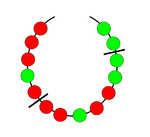Splitting a necklace with two cuts.

Combinatorial analogs of concepts and methods in topology are used to study graph coloring, fair division, partitions, partially ordered sets, decision trees, necklace problems and discrete Morse theory. It should not be confused with combinatorial topology which is an older name for algebraic topology.

### Arithmetic combinatorics

Arithmetic combinatorics arose out of the interplay between number theory, combinatorics, ergodic theory and harmonic analysis. It is about combinatorial estimates associated with arithmetic operations (addition, subtraction, multiplication, and division). Additive combinatorics refers to the special case when only the operations of addition and subtraction are involved. One important technique in arithmetic combinatorics is the ergodic theory of dynamical systems.

### Infinitary combinatorics

Infinitary combinatorics, or combinatorial set theory, is an extension of ideas in combinatorics to infinite sets. It is a part of set theory, an area of mathematical logic, but uses tools and ideas from both set theory and extremal combinatorics.

Gian-Carlo Rota used the name continuous combinatorics to describe probability and measure theory, since there are many analogies between counting and measure.

## Related fields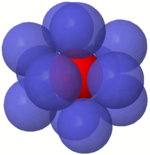Kissing spheres are connected to both coding theory and discrete geometry.

### Combinatorial optimization

Combinatorial optimization is the study of optimization on discrete and combinatorial objects. It started as a part of combinatorics and graph theory, but is now viewed as a branch of applied mathematics and computer science, related to operations research, algorithm theory and computational complexity theory.

### Coding theory

Coding theory started as a part of design theory with early combinatorial constructions of error-correcting codes. The main idea of the subject is to design efficient and reliable methods of data transmission. It is now a large field of study, part of information theory.

### Discrete and computational geometry

Discrete geometry (also called combinatorial geometry) also began a part of combinatorics, with early results on convex polytopes and kissing numbers. With the emergence of applications of discrete geometry to computational geometry, these two fields partially merged and became a separate field of study. There remain many connections with geometric and topological combinatorics, which themselves can be viewed as outgrowths of the early discrete geometry.

### Combinatorics and dynamical systems

Combinatorial aspects of dynamical systems is another emerging field. Here dynamical systems can be defined on combinatorial objects. See for example graph dynamical system.

### Combinatorics and physics

There are increasing interactions between combinatorics and physics, particularly statistical physics. Examples include an exact solution of the Ising model, and a connection between the Potts model on one hand, and the chromatic and Tutte polynomials on the other hand.

Wikimedia Foundation. 2010.

Нужна помощь с курсовой?

### Look at other dictionaries:

• combinatorics — [käm΄bə nə tôr′iks] n. a branch of mathematics dealing with combinations and permutations …   English World dictionary

• combinatorics — /keuhm buy neuh tawr iks, tor , kom beuh /, n. (used with singular v.) See combinatorial analysis. * * * Branch of mathematics concerned with the selection, arrangement, and combination of objects chosen from a finite set. The number of possible… …   Universalium

• combinatorics — noun plural but singular in construction Date: 1941 combinatorial mathematics …   New Collegiate Dictionary

• combinatorics — noun a branch of mathematics that studies (usually finite) collections of objects that satisfy specified criteria (see for further details) …   Wiktionary

• combinatorics — n. (Mathematics) branch of mathematics that deals with individual sets and problems of combination (divided into sub branches: combinatorial analysis, combinatorial geometry, etc.) …   English contemporary dictionary

• combinatorics — [ˌkɒmbɪnə tɒrɪks] plural noun [treated as sing.] the branch of mathematics dealing with combinations of objects belonging to a finite set in accordance with certain constraints …   English new terms dictionary

• combinatorics — com·bi·na·to·rics …   English syllables

• combinatorics — /kɒmbɪnəˈtɔriks/ (say kombinuh tawriks) noun → combinatorial analysis …   Australian-English dictionary

• combinatorics — ˌkämbə̇nəˈtōriks, ȯr also kəmˌbīn noun plural but singular in construction Etymology: combinatorial mathematics : combinatorial mathematics …   Useful english dictionary

• Combinatorics and physics — Combinatorial physics or physical combinatorics is the area of interaction between physics and combinatorics. Combinatorial Physics is an emerging area which unites combinatorial and discrete mathematical techniques applied to theoretical physics …   Wikipedia